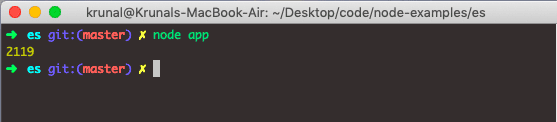# Javascript ParseInt: How to Use JavaScript parseInt() Function

1
17Javascript parseInt() is an inbuilt method that converts the string into an integer (a whole number). It accepts the two arguments. The first argument is the string, which needs to convert and the second argument is called the radix. Radix is the base number used in mathematical systems. JavaScript provides various ways to convert a string value into a number.

## Javascript ParseInt Example

Javascript parseInt() function parses a string argument and returns an integer of the specified radix (the base in mathematical numeral systems). The parseInt() function is standard Javascript built-in objects.

### Syntax

`parseInt(string, radix);`

### Parameters

The string parameter is required, and it is the argument that needs to be converted into an integer.

The radix parameter is optional, and it is an integer between 2 and 36 that represents the radix which is the base in mathematical numeral systems of the string mentioned above.

See the simple example of converting the string into an integer in Javascript.

```// app.js

let a = parseInt('21');
console.log(a);```

#### OutputThe parseInt function converts its first argument to the string, parses it, and returns an integer or NaN. If not NaN, then the returned value would be an integer.

See some more examples.

```// app.js

console.log(parseInt('0xF', 16));
console.log(parseInt('F', 16));
console.log(parseInt('17', 8));
console.log(parseInt(021, 8));
console.log(parseInt(15.99, 10));
console.log(parseInt('15,123', 10));
console.log(parseInt('FXX123', 16));
console.log(parseInt('1111', 2));
console.log(parseInt('15 * 3', 10));
console.log(parseInt('15e2', 10));
console.log(parseInt('15px', 10));
console.log(parseInt('12', 13));```

See the output below — all returns 15.But if the string does not start with a number, you’ll get NaN (Not a Number).

`console.log(parseInt("I am 21", 10));`

#### OutputThe Radix parameter is used to specify which numerical system will be used, for example, the radix of 16 (hexadecimal) indicates that a number in a string should be parsed from the hexadecimal number to the decimal number.

If a radix parameter is not specified, the javascript assumes the following.

• If the string begins with ‘0x’, the radix is 16 (hexadecimal).
• If the string begins with ‘0’, the radix is 8 (octal). This feature is deprecated.
• If a string begins with any other value, the radix is 10 (decimal).

Note that only the first number in the string is returned!

Note that Leading and trailing spaces are allowed.

Note that If the first character cannot be converted to a number, parseInt() returns NaN.

Note that Older browsers will result in parseInt(“010”) as 8, because older versions of ECMAScript, (older than ECMAScript 5, use the octal radix (8) as default when a string begins with “0”. As of ECMAScript 5, the default is the decimal radix (10).

There is also one other method in Javascript that can convert the string into an integer, and that is the Number object.

```// app.js

const count = Number('2119')
console.log(count);```

#### OutputFinally, Javascript ParseInt() Function example is over.

#### 1 Comment

1.Amit Parmar

thanks for the post

This site uses Akismet to reduce spam. Learn how your comment data is processed.